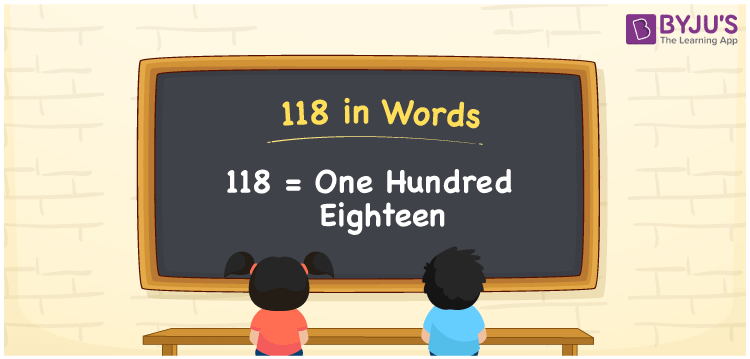# 118 in Words

118 in words is written as “One Hundred Eighteen”. For example, Maitri says “I have given Rupees One Hundred Eighteen to my friend”. In Mathematics, 118 is a natural number or an integer that is used to represent a value or a quantity. Learn to write the number 118 in words in this article, using the place value method and more about Numbers In Words at BYJU’S.

 118 in Words One Hundred Eighteen One Hundred Eighteen in Numerical Form 118

## 118 in English Words## How to Write 118 in Words?

118 in words is written based on the place value of each digit in the given number. Since 118 is a three-digit number, thus;

 Hundreds Tens Ones 1 1 8

From the above table, we can see that

• 1 is at Hundreds
• 1 is at Tens
• 8 is at Ones

We need to read the number from right to left, in the table. Hence, it is spelt as One Hundred Eighteen.

### Expanded Form of 118

We can write the expanded form of 118 as:

1 at Hundreds + 1 at Tens + 8 at Ones

= 1 × 100 + 1 × 10 + 8 × 1

= 100 + 10 + 8

= 100 + 18

= 118

= One Hundred Eighteen

118 is a whole number that is succeeded by 117 and preceded by 119. Find more about the number 118 below:

• 118 in Words – One Hundred Eighteen
• Is 118 an odd number? – No
• Is 118 an even number? – Yes
• Is 118 a perfect square number? – No
• Is 118 a perfect cube number? – No
• Is 118 a prime number? – No
• Is 118 a composite number? – Yes

## Frequently Asked Questions on 118 in words

Q1

### What is 118 in words?

118 in words is given by One Hundred Eighteen.
Q2

### What is the rule to write 118 in words?

118 in words is written based on the place values of each digit.

1 → Hundreds

1 → Tens

8 → Ones

Thus, the number is written as One Hundred Eighteen.

Q3

### What is the value of 118 + 100 in words?

The value of 118 + 100 = 218. It is written as Two Hundred Eighteen in the form of words.
Test your Knowledge on 118 in Words